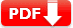# ACT Math Practice Test 2 (60 Question Full Test with PDF and Solution)

ACT Math Practice Test 2 (60 Question Full Test with PDF and Solution): Free printable PDF and editable doc file available for this Math practice test ACT exam.

## ACT Math Practice Test 2

Test Name ACT Test Prep Free ACT Practice Test Algebra, Geometry, and Trigonometry Sample Multiple Choice either join the free full-length test or review question and solution 60 60 minute (1 hour) YES YES YES

## Online ACT Math Practice Test 2FREE ACT Full Math Practice Test – 2

60 Questions—60 Minutes

Click on the START button

## ACT Math Practice Test with Explanation

Direction: For each problem, choose the correct answer. You are allowed to use a calculator on this test for any problems you choose. Unless the problem states otherwise, you should assume that figures are not drawn to scale. For this test, all geometric figures lie in a plane, the word line refers to a straight line, and the word average refers to the arithmetic mean.

Q1. How is five hundred twelve and sixteen-thousandths written in decimal form?

• A). 512.016
• B). 512.16
• C). 512,160
• D). 51.216
• E). 512.0016

Solution: The word and indicates a decimal point. Therefore, the decimal point should go after 512 and before 16 thousandths. The number 16 must end in the thousandths place, which is three digits to the right of the decimal

Q2. Simplify |3 − 11| + 4 × 23

• A). 421
• B). 520
• C). -10
• D). 40
• E). 28

Solution: The correct order of operations must be used to simplify the expression. You may remember this as PEMDAS: parentheses, exponents, multiplication, division, addition, subtraction.

Q3. The ratio of boys to girls in a math class is 4 to 5. If there are 18 students in the class, how many are boys?

• A). 2
• B). 4
• C). 8
• D). 12
• E). 13
Solution:  The problem can also be worked with the equation 4x + 5x = 18, where x equals each unit when there are 4 units of boys and 5units of girls.

Q4. What is the median of 0.024, 0.008, 0.1, 0.024, 0.095, and 0.3?

• A). 0.0595
• B). 0.0969
• C). 0.055
• D). 0.0566
• E). 0.95

Solution:  To find the median, place the numbers in order from least to greatest and find the middle number. Because there are an even
number of numbers, there are two middle numbers (0.024 and 0.095). Take the average of these two middle numbers.

Q5. Which of the following is NOT the graph of a function?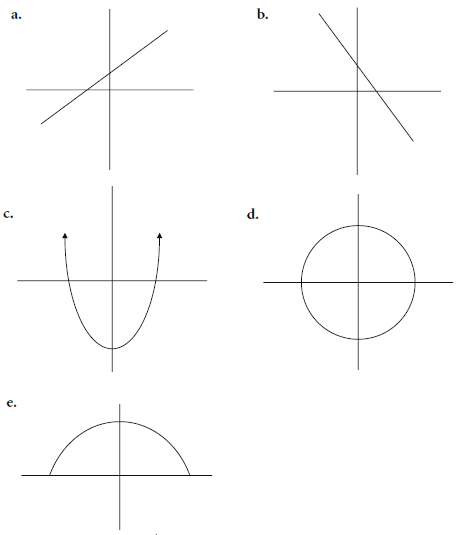Solution: Use the vertical line test to see if each graph is a function. A graph is not a function if any vertical line drawn goes through the graph more than once (if there is more than one y value for any x value).

Q6. What is the value of x5 for x = –3?

• A). –243
• B). 243
• C). –43
• D). 43
• E). 13

Solution:  –3 × –3 × –3 × –3 × –3 = –243

Q7. What is the next number in the following pattern? 0, 3, 8, 15, 24, . . .

• A). 34
• B). 35
• C). 36
• D). 37
• E). 33

Solution:  Consecutive odd integers starting with 3 are being added to find the next number. Therefore, 11 must be added to 24 to find the next number. The answer is 35

Q8. What is the prime factorization of 84?

• A). 42 × 2
• B). 7 × 2 × 3
• C). 22 × 3 × 7
• D). 22 × 6 × 7
• E). 23 × 7

Solution:  First, you can eliminate choices f and j because they contain numbers that are not prime. Next, use a factor tree to determine
the prime factorization. The prime factorization of 84 is 2 × 2 × 3 × 7, which can be written in exponential notation as 22 × 3 × 7.

Q9. Find the slope of the line 7x = 3y − 9.

• A). 3
• B). −9
• C). 3/7
• D). −3
• E). 7/3

Solution:  o easily see the slope, change the equation into the form y = mx + b. The equation is then y = (z/3) x + 9, where m is the slope

Q10. The perimeter of a rectangle is 20 cm. If the width is 4 cm, find the length of the rectangle.

• A). 5 cm
• B). 6 cm
• C). 15 cm
• D). 25 cm
• E). 32 cm

Solution:  The perimeter is twice the width plus twice the length: P = 2w + 2l. Insert 20 for P and 4 for w, then solve for l.

Q11. Find the area of the following figure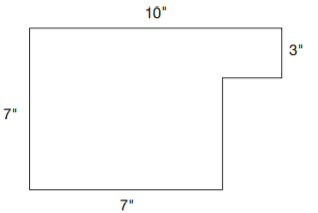• A). 58 sq. in.
• B). 18 sq. in.
• C). 51 sq. in.
• D). 39 sq. in.
• E). 98 sq. in.

Solution:  Find the lengths of the unlabeled sides by comparing them to the given sides. Find the area of each of the regions and add together to find the total area: 30 + 28 = 58 sq. in.

Q12. Five cans of tomatoes cost $6.50. At this rate, how much will nine cans of tomatoes cost? • A).$13.00
• B). $11.70 • C).$3.30
• D). $4.70 • E).$13.70
Solution:  Find the cost for one can (unit rate) by dividing the cost of five cans by 5: $6.50 ÷ 5 =$1.30 per can. Multiply the cost per can
by 9 cans: $1.30 × 9 =$11.70. Nine cans cost \$11.70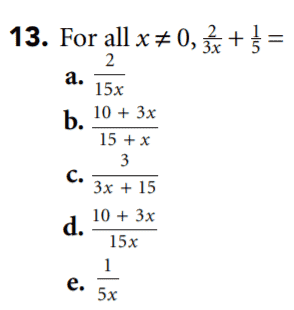Solution: . Find a common denominator (15x). Multiply the numerator and denominator of the first fraction by 5 and of the second fraction
by 3x; then add the two numerators and place them over the common denominator.

Q14. Which inequality best represents the following graph?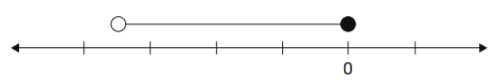• A). −1.5 > x > −1
• B). x ≤ 0
• C). −0.5 > x > 0
• D). −1.5 < x ≤ 0
• E). −1.5 ≤ x ≤ 0
Solution:  The endpoints are on −1.5 and 0, and the solution set includes 0 but does not include –1.5, as indicated by the open circle.

Q15. Simplify −(6x4y3)2

• A). −36x6y5
• B). −36x8y6
• C). 366x22y
• D). −36xy
• E).  −36x2y
Solution: First, raise everything in the parentheses to the second power; then apply the negative.

Q16. If 2x + 3y = 55 and 4x = y + 47, find x − y

• A). 6
• B). 12
• C). 24
• D). 28
• E). 32
Solution:  Use substitution to solve for x and y. First, solve the second equation for y. Next, substitute the value for y into the first equation
and solve for x. Finally, subtract y from x.

Q17. Simplify  3\sqrt{16x^5y^4}

• A). 2xy 3\sqrt{2x^2}
• B). 8xy 3\sqrt{2}
• C).  2xy 3\sqrt{xy}
• D). 2x2y
• E). 4x2y2
Solution: Notice that you are taking the cube root, not the square root. Break up the expression under the radical into perfect cubes.

Q18. The formula to convert Celsius to Fahrenheit is  where F= \frac95 C + 32 is degrees Fahrenheit, and C is degrees Celsius. What Fahrenheit temperature is equivalent to 63°C?

• A). 35°
• B). 49°
• C). 67°
• D). 89°
• E). 97°
Solution: Substitute the value 63° for C, and solve for F

Q19. What are the solutions to the equation x2 + 8x + 15 = 0?

• A). {8,15}
• B). {0}
• C). {−5,−3}
• D). {−8,−13}
• E). {2,4}
Solution:  The equation is quadratic. Because it is factorable, solve the equation using factoring: (x + 5)(x + 3) = 0. Set each of the factors
equal to zero and solve for x: x + 5 = 0, x + 3 = 0, so x = –5 and x = –3.

Q20. If 5k = 9m − 18, then m =

• A). 5k + 18
• B). (5k+18) / 9
• C). −9 + 5k
• D). (5k + 9 ) / 9
• E). 9k + 18
Solution:  Solve the equation for m using inverse operations

Q21. What are the solutions of the equation 5x − 7 = 5(x + 2)?

• A). {2}
• B). {7}
• C). all positive numbers
• D). all real numbers
• E). empty set
Solution:  By distributing and then using inverse operations, you get the equation –7 = 10, so there are no values for x that make the equation true.

Q22. \frac{4x^2 + 11x - 3} {x+3} for all x ≠ −3

• A). 3x2 + 11
• B). 2x + 1
• C). 4x2 + 1
• D). 10x − 6
• E). 4x − 1
Solution:  Factor the numerator into (4x – 1)(x + 3). Cancel out the (x + 3) in numerator and denominator, and 4x – 1 remains.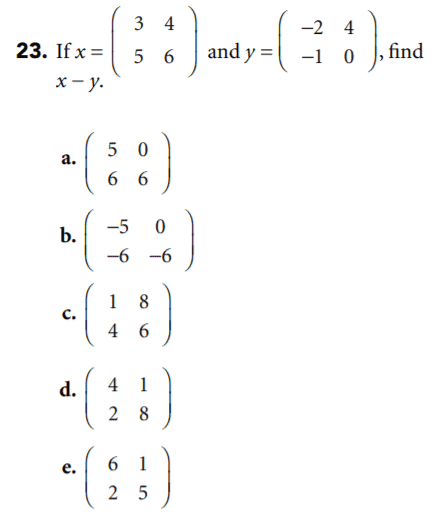Solution:  Subtract the numbers in y from the corresponding numbers in x.

Q24.  If log3x = 2, then x =

• A). 6
• B). 9
• C). ¼
• D). ½
• E). ¾
Solution: Log3x = 2 is equivalent to 32 = x. Therefore, x = 9

Q25. The vertices of a triangle are A(−1,3), B(3,0), and C(−2,−1). Find the length of side AC.

• A). √15
• B). √17
• C). 1√5
• D). √7
• E). 1√7
Solution:  Use the Pythagorean theorem to solve. To use the Pythagorean theorem (which is what the distance formula is derived from), draw the segment on a coordinate plane and create a right triangle where AC is the hypotenuse. The legs of the right triangle are 1 and 4, so the hypotenuse is the square root of 12 + 42, or the square root of 17.

Q26.  Which of the following equations has a graph that has a y-intercept of 4 and is parallel to 3y − 9x = 24?

• A). −12x + 4y = 16
• B). 9x − 3y = −15
• C). 2y = 4x + 8
• D). 7y = 14x + 7
• E). 3x − 9y = 14
Solution:  Because the line is parallel to 3y – 9x = 24, they must have the same slope. Put the equation into y = mx + b form to see the slope is 3 and the y-intercept is 4, so you are looking for the line y = 3x + 4. You must put the answer choices in y = mx + b form to compare them to this equation

Q27.  The best approximation of \sqrt{37} × \sqrt{125}

• A). 52
• B). 1058
• C). 2566
• D). 138
• E). 66
Solution:  The answer choices are spread out enough that a quick bit of figuring establishes the useful range. Use the square roots that are
near the answer. For 37, use 6; for 125, use 11. These will quickly lead to 66 as the correct choice

Q28. If a coin is flipped and a number cube is rolled, what is the probability of getting tails and 3?

• A). ¼
• B). ½
• C). ¾
• D). 1/12
• E). 2/10
Solution:  Find the probability of each event and multiply the answers to find the probability of both events occurring. The probability of
getting tails is 1/2, and the probability of rolling a 3 is 1/6.

Q29. What is ½% of 90?

• A). 0.04
• B). 0.145
• C). 45
• D). 0.45
• E). 145
Solution:  Multiply the decimal equivalent of ½ %, or 0.005, by 90.

Q30. Mike has 12 bags of shredded cheese for making pizzas. If he uses ¾ of a bag of cheese for each pizza, how many pizzas can he make?

• A). 6
• B). 8
• C). 16
• D). 32
• E). 49
Solution: Set up a proportion of (0.75/1) = (12/x) , cross multiply, and solve for x.

Q31. Greene ran the 100-meter dash in 9.79 seconds. What was his speed in km/h (rounded to the nearest km)?

• A). 3 km/h
• B). 7 km/h
• C). 17 km/h
• D). 37 km/h
• E). 47 km/h
Solution:  Convert the given rate of meters per second to kilometers per hour. First, multiply (100/9.75) by (1/1000) to change meters to kilometers. Next, multiply that product by 60/1 to change seconds to minutes. Finally, multiply that product by 60/1 to changes minutes to hours.

Q32. Larry has 4 pairs of pants, 6 pairs of socks, and 10 shirts in his dresser. How many days could Larry go without wearing the same combination of 1 pair of pants, 1 pair of socks, and 1 shirt?

• A). 5
• B). 52
• C). 152
• D). 382
• E). 452
Solution:  To find the number of combinations available, multiply the number of choices for each element, or 4 × 6 × 10, which is 240.

Q33. What is the product of 5 × 10−4 and 6 × 108?

• A). 3 × 105
• B). 7 × 104
• C). 1.1 × 10-5
• D). 3.6 × 10-4
• E). 5.6 × 104
Solution:  Multiply the 5 by 6 to get 30. Then multiply the powers of 10 to get 104, or 10,000. 30 × 10,000 = 300,000. Now convert to scientific notation.

Q34. What is the sine of angle B in the triangle?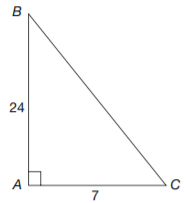• A). 7/25
• B). 5/27
• C). 9/28
• D). 3/ 37
• E). 4/39
Solution: sin = opposite/hypotenuse, so you must find the hypotenuse. To do so, use the Pythagorean theorem (or simply recognize the
Pythagorean triple). The hypotenuse is 25, so sin B = 7/25 .

Q35. If the surface area of a box is found by taking the sum of the areas of each of the faces of the box, what is the surface area of a box with dimensions 6 in. by 8 in. by 10 in.?

• A). 76 sq. in.
• B). 176 sq. in.
• C). 276 sq. in.
• D). 376 sq. in.
• E). 476 sq. in.
Solution:  There are three pairs of sides. The two sides that measure 6 × 8 each have an area of 48. The two sides that measure 6 × 10 each have an area of 60. The two sides that measure 8 × 10 each have an area of 80. Simply multiply and add.

Q36. Find the area of the shaded region.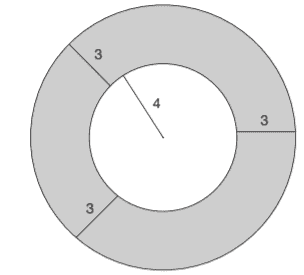• A). 6π
• B). 16π
• C). 33π
• D). 62π
• E). 77π
Solution:  The radius of the large circle is 7 (add the inner radius plus the extra 3 from the ring); therefore the area of the large circle is 49π.
Subtract the area of the inner circle, which is 16π

Q37. The area of square WXYZ is 100 sq. cm. Find the length of diagonal WY in cm.

• A). 10 √2 cm
• B). 20 √2 cm
• C). 10 cm
• D). 15 cm
• E).  10 √5 cm
Solution: Take the square root of the area to find the length of one side, 10. When the diagonal is drawn, it creates a right triangle with legs of 10 cm each and the diagonal is the hypotenuse. Use the Pythagorean theorem to find the diagonal.

Q38. Which of the following could be the value of x when y is equal to 15 for the equation y = 4×2 − 1?

• A). –1
• B). –2
• C). –4
• D). 0
• E). √2
Solution: Substitute 15 for y in the equation and solve for x

Q39. At Roosevelt High, Kristen won the election for class president with 60% of the vote. Of that 60%, 75% were female. If 540 students voted, how many female students voted for Kristen?

• A). 149
• B). 243
• C). 278
• D). 313
• E). 416
Solution:  Find the number of students (male and female) who voted for Kristen by multiplying 540 by 0.60, the decimal equivalent of
60%. Multiply that product by 0.75, the decimal equivalent of 75%

Q40. If cos θ = 12/13 and tan θ = 5/12 , then sin θ =

• A). 5/13
• B). 7/9
• C). 5/11
• D). 12/5
• E). 13/5
Solution:  cos is adjacent/hypotenuse, and tan is opposite/adjacent. If cos θ is 12/13, then the hypotenuse is 13. The side opposite θ is 5, so
sin θ = (opposite/ hypotenuse) = 5/13

Q41. The formula for the volume of a rectangular solid is V = lwh. If each dimension is tripled, how many times the original volume will the new volume be?

• A). 3
• B). 9
• C). 27
• D). 89
• E). 173
Solution: The original formula was V = lwh. If each dimension is tripled, the length is 3l, the width is 3w, and the height is 3h. When these
values are substituted into the equation, the equation becomes V = (3l)(3w)(3h), or V = 27lwh. Thus the new rectangular solid has a volume 27 times the original volume.

Q42. In a right triangle, the two non-right angles measure 7x and 8x. What is the measure of the smaller angle?

• A). 15°
• B). 27°
• C). 42°
• D). 49°
• E). 61°
Solution:  The sum of the measures of the angles in a triangle is 180°. In a right triangle, the right angle is 90°, so another 90° is split between the remaining two angles. Thus 7x + 8x = 90, and x = 6. The value of x is 6, but the question asks for the measure of the smaller
angle, which is 7x, or 42.

Q43. The length of a rectangle is twice the width. If the perimeter of the rectangle is 72 feet, what is the length of the rectangle?

• A). 3
• B). 7
• C). 12
• D). 24
• E). 32
Solution: Call the width w and the length 2w. The perimeter is then P = w + w + 2w + 2w; 72 = 6w; 12 = w. The width is 12. Because the length is twice the width, the length is 24

Q44. The area of a triangle is 80 sq. in. Find the height if the base is 5 in. more than the height.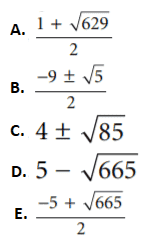Q45. Three of the vertices of a square are (−2,3), (5,3), and (−2,−4).What is the length of a side of the square?

• A). 4
• B). 5
• C). 6
• D). 7
• E). 9
Solution:  There is no need to use the distance formula if you recognize that the y-coordinate of the first two points is the same (3). This means that the difference in the x-coordinates, which is 7, must be the length of one side of the square.

Q46.  Which of the following lines is perpendicular to y = 3x + 1?

• A). f. 6x + 5 = 2y
• B). 4 + y = 3x
• C). −9y = −3 + 2x
• D). 2x + y = 4
• E).3 y + x = 5
Solution: The slope of the given equation is 3. The slope of a line perpendicular to the line is the negative reciprocal of 3, or – (1/3) Arrange each answer choice into the y = mx + b format to find the slope of each choice quickly. Only choice k has the proper slope.

Q47. What is the midpoint of line XY if X(−4,−2) and Y(3,8)?

• A). (−7,6)
• B). (−0.5,3)
• C). (−1,6)
• D). (−7,−10)
• E). (2,−1.5)
Solution:  To find the midpoint, use the midpoint formula, which in effect means find the averages of the two x-coordinates and the two
y-coordinates

Q48. Simplify (½ x2)-3

• A). 6x3
• B). 8x6
• C). (8/x6)
• D). 8x
• E). 6x
Solution: Apply the exponent to both ½ and x2. Because the exponent is negative, take the reciprocal of the fraction and the variable,
then raise both 2 and 1/x2 to the third power and combine.

Q49. Line l is parallel to line m. Find the measure of angle x.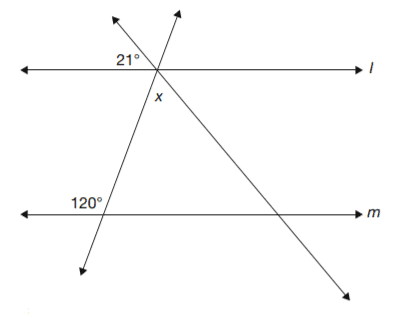• A). 21°
• B). 39°
• C). 99°
• D). 106°
• E). 121°
Solution: Recall that all triangles have three angles that total 180°. Next, using the two angle measures given, find the two bottom angles of
the triangle. The bottom left angle is supplementary (adds to 180°) with 120°; therefore, it is 60°. The bottom right angle is a corresponding angle to the 21° angle and, therefore, is 21°. The three angles in the triangle must add to 180°, so x is 99°

Q50. Find the radius of the circle with center (4,−2) that is tangent to the y-axis

• A). 2
• B). 6
• C). 1
• D). 4
• E). 10
Solution: Keep in mind that a tangent line will have only one point in common with the circle. Draw the graph of the circle on the coordinate plane to see that the radius must be 4.

Q51. Find the area, in square units, of the circle shown by the equation (x − 5)2 + (y − 2)2 = 36

• A). 4π
• B). 25π
• C). 25π
• D). 36π
• E). 48π
Solution:  The equation of a circle is in the form (x −h)2 + (y −k)2 = r2, where r is the radius. Because the given equation is already in this form, you can find that r2 = 36, or r = 6. Use the formula A = πr2 to find the area of the circle.

Q52. m ∠ABC = 120° and m ∠CDE = 110°. Find the measure of ∠C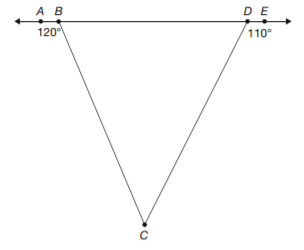• A). 21°
• B). 39°
• C). 99°
• D). 106°
• E). 121°
Solution:  Find the measures of angles DBC and BDC by using the supplements given (remember that supplementary angles add to 180°). So angle DBC = 60° and angle BDC = 70°. The three angles of a triangle must add to 180°. Therefore, angle BCD = 50°.

Q53.  What is the minimum value of 9 cos x?

• A).a. –9
• B). –2
• C). 0
• D). 2
• E). 9
Solution:  The cosine graph goes from –1 to 1, meaning the cosine of an angle is between –1 and 1, inclusive, and the minimal value for cos x
is –1. Now multiply by 9 to solve.

Q54. The Abrams put a cement walkway around their rectangular pool. The pool’s dimensions are 12 ft. by 24 ft., and the width of the walkway is 5 ft. in all places. The area of the walkway is

• A). 205 sq. ft.
• B). 288 sq. ft.
• C). 460 sq. ft.
• D). 493 sq. ft.
• E). 748 sq. ft.
Solution:  The walkway is 10 ft. longer and 10 ft. wider than the pool (not 5 ft.) because 5 ft. is added on each side of the pool. To find the
area of the walkway, find the area of the large rectangle (walkway and pool combined), and subtract the area of the pool

Q55. Triangle XYZ is an equilateral triangle. \overline{\rm YW} is an altitude of the triangle. If \overline{\rm XY} is 10 in., what is the length of the altitude?

• A). 5 in.
• B). 5 √3 in.
• C). 10 in.
• D). 10 √3 in.
• E). 5√2 in.
Solution: Equilateral triangle XYZ has all 60° angles. Because YW is an altitude, it forms a right angle and bisects the angle at Y. Thus, triangle XYW is a 30–60–90 triangle. The hypotenuse YX is 10 in., and the shortest side XW is half of that, 5 in. The side opposite the 60°
angle is √3 times the short side

Q56. Find the value of cos A if angle A is acute and sin A = 7/10

• A). 3/10
• B). 1/9
• C). 7/√51
• D). 51/10
• E). √51/10
Solution:  Use the identity sin2A + cos2A = 1, so 49/100 + cos2A = 1. Now solve by subtracting 49/100 from both sides, then taking the square root of both sides

Q57. Find the value of x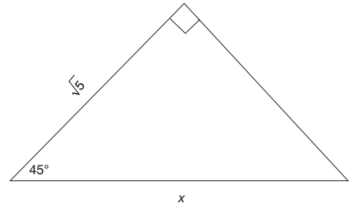• A). 2
• B). 1
• C). √7
• D). √10
• E). 2 √5
Solution: The triangle given is a 45–45–90 triangle, so the sides are in the ratio 1:1: 2. Use a proportion to find x, where (√2/1) = (x/ √5)

Q58. Which equation corresponds to the graph?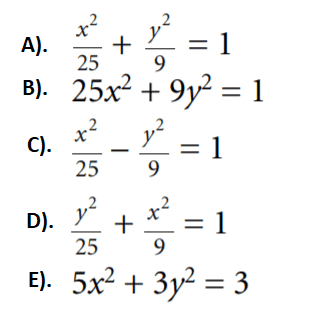Q59. What is the inequality that corresponds to the graph?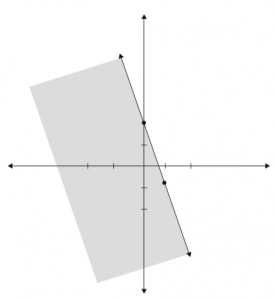• A). y > 3x + 2
• B). y ≤ −3x + 2
• C). y ≥ −3x + 2
• D). y < 3x + 2
• E). y < −3x + 2
Solution: Notice that the y-intercept is 2 and the slope is −3. Thus the equation must be y = −3x + 2. Answer choices b and c are possibilities. The shading determines which is correct. Substitute (0,0) for x and y. Because the shading includes the point (0,0), find the
equation that is satisfied by the point (0,0).

Q60.  What is the domain of the function f (x) =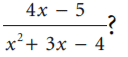• A). {x | x ≠ 0}
• B). Ø
• C). all real numbers
• D). {x | x ≠ 3}
• E). {x | x ≠ −4 and x ≠ 1}
 Document Type Download Link Free Editable Doc File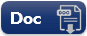Free Printable PDF File